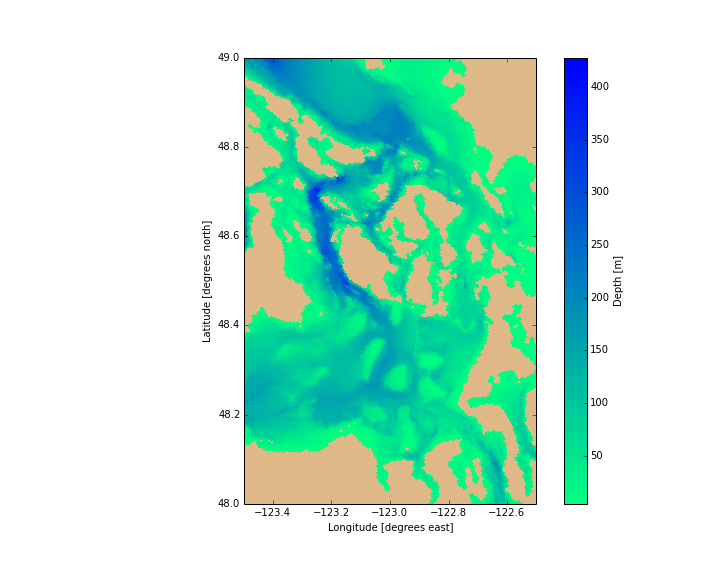# Plotting Bathymetry Colour Meshes¶

This notebook contains discussion, examples, and best practices for plotting bathymetry data as colour meshes. It also serves as a basic introduction setting up horizontal slice visualizations of any model result quantities. Topics include:

• The matplotlib library and its pyplot and pylab interfaces
• The salishsea_tools.viz_tools code module
• Making plots appear below the code cells that create them in Jupyter Notebooks
• Figure and Axes objects
• Plotting bathymetry depth data as 2-D pseudocolour mesh plots
• Controlling the size and aspect ratio of plots, in particular setting the aspect ratio to match the Salish Sea NEMO model lateral grid
• Using different colour maps for colour mesh plots and adding colour bar scales to them
• Controlling the colour of land areas
• Setting axes limits and zooming in on regions of the domain
• Plotting on latitude/longitude map coordinates
• Saving plots as image files and displaying image files in notebooks

The matplotlib library is our primary visualization tool. It's basic interface is quite similar to Matlab's plotting commands, but it is built on top of an object-oriented, Pythonic API. Matplotlib is well integrated with IPython and Jupyter Notebook, allowing plots to be updated on the fly as code is changed, and displaying them with notebooks. It also provides means to save plot figures in a variety of bitmap and vector formats including: PNG, JPG, PDF, PS, and SVG.

There are several ways of importing and using matplotlib and its various components. We will focus on the matplotlib.pyplot interface. In the Matplotlib docs and elsewhere on the Internet you will often see examples that use the pylab interface. Quoting from the matplotlib docs:

Pyplot provides the state-machine interface to the underlying plotting library in matplotlib.
This means that figures and axes are implicitly and automatically created to achieve the desired plot.
...
Pylab combines the pyplot functionality (for plotting) with the numpy functionality
(for mathematics and for working with arrays) in a single namespace,
making that namespace (or environment) even more MATLAB-like.



So, you can use the same method calls that you seen in pylab examples with the pyplot interface because pylab is just a superset of pyplot. In the spirit of the "Explicit is better than implicit." aphorisms in the Zen of Python we'll keep references to pyplot and NumPy explicit and separate.

In :
from __future__ import division, print_function

import matplotlib.pyplot as plt
import netCDF4 as nc

from salishsea_tools import viz_tools


We have also imported the salishsea_tools.viz_tools code module, a collection of functions for doing routine tasks associated with plotting and visualization. It will be introduced and used below.

We also want to activate the Jupyter Notebook %matplotlib magic. Without that our plot won't be rendered at all (or they may be rendered in a separate window, depending on your operating system). To make the plot appear below the code cells that create them in our notebook we specify the inline backend:

In :
%matplotlib inline


Let's get some data to look at by loading the depths array from the Salish Sea NEMO model bathymetry file. (For an introduction to working with netCDF files see the Exploring netCDF Files notebook.)

In :
grid = nc.Dataset('../../NEMO-forcing/grid/bathy_meter_SalishSea2.nc')
bathy = grid.variables['Bathymetry'][:]


Note that we're assigning the depths data array to our bathy variable by way of the [:] slice notation because we're really interested in the depths and not the netCDF variable features. Doing the "whole array" slice now saves us from having to type it repeatedly later.

## Figures and Axes¶

To be able to control things like the aspect ratio of our plots and the size of the colour bar relative to the plot area we need access to the Figure and Axes object instances that pyplot creates. plt.subplots() (note the s on the end) will create and return those instances:

In :
fig, ax = plt.subplots(1, 1, figsize=(10, 8))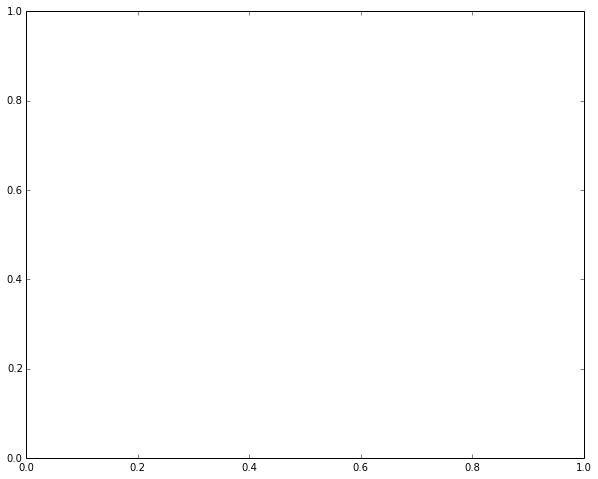We'll see in the Plotting Salinity and Temperature notebook how to use the first 2 arguments to plt.subplots() plot multiple sub-plots in the same figure. For now we just use 1, 1 to get a single sub-plot. The figsize argument sets the size and shape of the overall figure object. The dimensions are nominally inches but in the context of a notebook they just control relative size. The order of the tuple is (width, height), so figsize also plays a role in controlling the aspect ratio of the plot. The figure instance (fig) is an invisible container around the plot axes (ax) that you see above. The figure is a matplotlib.figure.Figure object instance, and the axes is a matplotlib.axes.Axes object instance.

## Colour Mesh Plots¶

We can plot our bathymetry data as a 2-D pseudocolour plot with the pcolormesh method. pcolormesh is preferred over pcolor because it is much faster, especially for large arrays (like our nearly 360,000 depth points). In addition, its point by point colouring reminds us of our grid resolution.

In :
fig, ax = plt.subplots(1, 1, figsize=(10, 8))
ax.pcolormesh(bathy)

Out:
<matplotlib.collections.QuadMesh at 0x10657d3d0>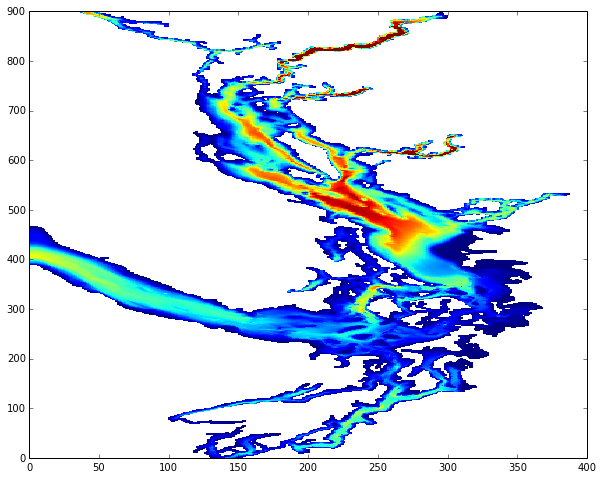There are several things to notice here:

• pcolormesh automatically used the size of our bathy array to plot the depths on lateral grid coordinates. We'll see later how to pass in latitudes and longitudes to plot on map coordinates.
• The land areas are white. That happened because bathy is a NumPy Masked Array. We can control the colour used to plot masked values.
• The plot is horribly distorted compared a map of the Salish Sea region.

## Aspect Ratio¶

Let's fix the distortion first by using the set_aspect method on our axes:

In :
fig, ax = plt.subplots(1, 1, figsize=(10, 8))
ax.set_aspect(5/4.4)
ax.pcolormesh(bathy)

Out:
<matplotlib.collections.QuadMesh at 0x1058cd210>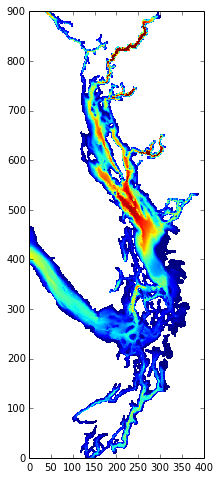The 5/4.4 aspect ratio is from the nominal 500 m by 440 m lateral size of our grid cells. The exact lateral cell size varies over the domain, but 5/4.4 is a good compromise. Fixing the aspect ratio of the axes made it occupy a much smaller portion of the figure area, so it appears smaller. If you have the vertical screen space (or don't mind scrolling through the axes) you can increase the size by increasing the height of the figure in the figsize argument.

If you are zooming in on a particular region of the domain and want to get more exact about the aspect ratio the following measurements are helpful:

• Near the centre of the domain cells (400, 200) $\rightarrow$ (400, 210) span an x-direction distance of 4.356 km, and (400, 200) $\uparrow$ (410, 200) span a y-direction distance of 5.021 km, hence the nominal 5/4.4 aspect ratio
• Near the centre of the bottom edge of the domain cells (0, 200) $\rightarrow$ (0, 210) span an x-direction distance of 4.519 km, and (0, 200) $\uparrow$ (10, 200) span a y-direction distance of 5.077 km
• Near the centre of the top edge of the domain cells (897, 200) $\rightarrow$ (897, 210) span an x-direction distance of 4.155 km, and (897, 200) $\downarrow$ (887, 200) span a y-direction distance of 4.872 km

The salishsea_tools.viz_tools.set_aspect() function is a thin wrapper around the Axes.set_aspect() method that sets the aspect ratio to 5/4.4 by default so that you don't have to remember that. It takes an axes object as its argument and you can also pass in an aspect ratio different from the default if you want/need to.

In :
help(viz_tools.set_aspect)

Help on function set_aspect in module salishsea_tools.viz_tools:

Set the aspect ratio for the axes.

This is a thin wrapper on the :py:meth:matplotlib.axes.Axes.set_aspect
method.
Its primary purpose is to free the user from needing to remember the
5/4.4 nominal aspect ratio for the Salish Sea NEMO model grid,
and the formula for the aspect ratio based on latitude for map
coordinates.
It also sets the axes aspect ratio with :py:attr:adjustable='box-forced'
so that the axes can be shared if desired.

:arg axes: Axes instance to set the aspect ratio of.
:type axes: :py:class:matplotlib.axes.Axes

:arg aspect: Aspect ratio.
:type aspect: float

:arg coords: Type of plot coordinates to set the aspect ratio for;
either :kbd:grid (the default) or :kbd:map.
:type coords: str

:arg lats: Array of latitude values to calculate the aspect ratio
from; only required when :kbd:coordinates='map'.
:type lats: :py:class:numpy.ndarray

:returns: Aspect ratio.
:rtype: float

.. note::

To explicitly set the aspect ratio for map coordinates
(instead of calculating it from latitudes)
set :kbd:aspect to the aspect ratio,
:kbd:coords='map',
and use the default :kbd:lats=None.



# Colour Bars and Colour Maps¶

Now let's add a colour bar to our figure to provide a scale for the depths:

In :
fig, ax = plt.subplots(1, 1, figsize=(10, 8))
viz_tools.set_aspect(ax)
mesh = ax.pcolormesh(bathy)
fig.colorbar(mesh)

Out:
<matplotlib.colorbar.Colorbar instance at 0x107afe560>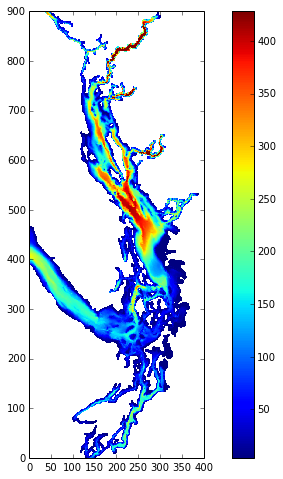The mesh object returned by the pcolormesh method provides the mapping between colours and depths for our colour bar. Calling the colorbar method on our figure object causes the colour bar to be added to the figure. The colour bar is actually a 2nd axes object within our figure object.

Matplotlib has lots of built-in colour maps that you can use, as well as providing methods that enable you to create your own. The colour map is specified via the cmap argument in the pcolormesh call. Versions of any of the built-in colour maps with their colour order reversed are obtained by appending _r to the colour map name. The reversed version of the winter colour map provides nice bathymetry colouring that is evocative of the productive shallow water and the darker, clearer deep water.

In :
fig, ax = plt.subplots(1, 1, figsize=(10, 8))
viz_tools.set_aspect(ax)
mesh = ax.pcolormesh(bathy, cmap='winter_r')
fig.colorbar(mesh)

Out:
<matplotlib.colorbar.Colorbar instance at 0x109319050>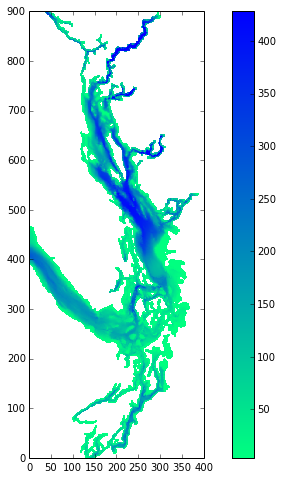# Land Area Colour¶

As noted above, the land areas are white because the bathymetry depths array is a NumPy masked array (see the Exploring netCDF Files notebook). We can change the colour used to plot masked values by manipulating the colour map. To do so we use the plt.get_cmap() function to return a cmap object instead of specifying the colour map by name in the pcolormesh() call. Once we have a cmap object we can use its set_bad() method to specify the colour to use to plot masked values. The colour can be given in at least 2 ways:

• by name as a string; e.g. 'burlywood', where any HTML colour name is valid
• by hex code as a string starting with #; e.g. '#edc9af'
In :
fig, ax = plt.subplots(1, 1, figsize=(10, 8))
viz_tools.set_aspect(ax)
cmap = plt.get_cmap('winter_r')
mesh = ax.pcolormesh(bathy, cmap=cmap)
fig.colorbar(mesh)

Out:
<matplotlib.colorbar.Colorbar instance at 0x10a5b4a28>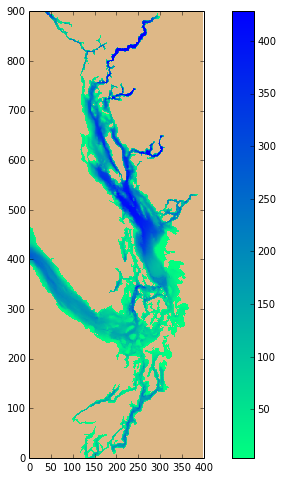The white strip down the right edge of the plot is a result of round-off by the matplotlib's auto-scaling functionality. We can avoid it by plotting with our axis limits set our actual data limits:

In :
fig, ax = plt.subplots(1, 1, figsize=(10, 8))
viz_tools.set_aspect(ax)
cmap = plt.get_cmap('winter_r')
mesh = ax.pcolormesh(bathy, cmap=cmap)
fig.colorbar(mesh)
plt.axis((0, bathy.shape, 0, bathy.shape))

Out:
(0, 398, 0, 898)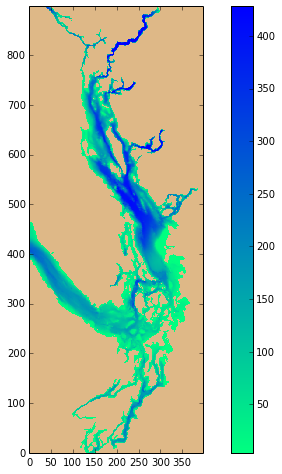The plt.axis() method is a short-cut that lets you set the x and y axis limits at once by passing is a 4-tuple like (xmin, xmax, ymin, ymax). You can also set the x and y axis limits individually with the ax.set_xlim() and ax.set_ylim() methods.

## Zooming In¶

The plt.axis() method can also be used to zoom in on particuar region:

In :
fig, ax = plt.subplots(1, 1, figsize=(10, 8))
viz_tools.set_aspect(ax)
cmap = plt.get_cmap('winter_r')
mesh = ax.pcolormesh(bathy, cmap=cmap)
fig.colorbar(mesh)
plt.axis((200, bathy.shape, 250, 400))

Out:
(200, 398, 250, 400)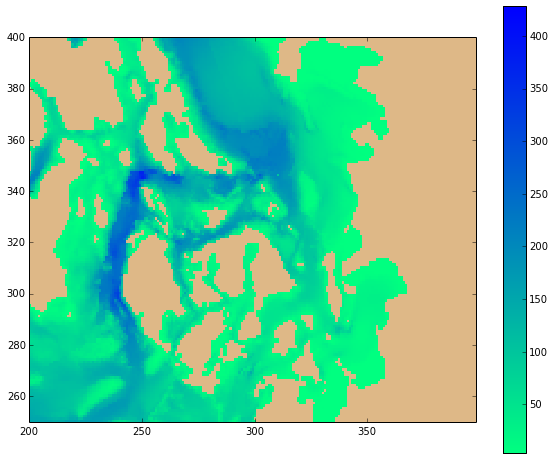Recall that our bathymetry plot and the colour bar are 2 distinct axes objects contained in our fig object. Setting the axis limits has changed the height of our bathymetry axes but the colour bar axes has stayed the full height of the fig. We can fix that with the shrink argument to the fig.colorbar() method:

In :
fig, ax = plt.subplots(1, 1, figsize=(10, 8))
viz_tools.set_aspect(ax)
cmap = plt.get_cmap('winter_r')
mesh = ax.pcolormesh(bathy, cmap=cmap)
fig.colorbar(mesh, shrink=0.85)
plt.axis((200, bathy.shape, 250, 400))

Out:
(200, 398, 250, 400)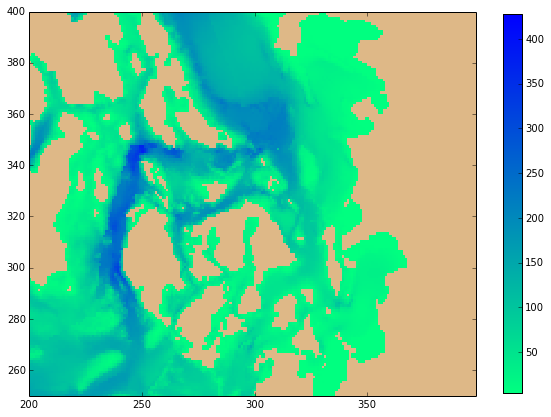In general, you will have to play with the figure size, axes limits, colour bar shrink value (and aspect ratio, if you change it) to get nicely proportioned looking plots.

## Plotting on Lat/Long Map Coordinates¶

We can also plot our bathymetry on latitude/longitude map coordinates. To do so we use the nav_lat and nav_lon variables from the netCDF file. We'll also re-define our bathy variable for this example so that we can make use of the netCDF attributes of the variables for aspect ratio and labeling.

In :
lats = grid.variables['nav_lat']
lons = grid.variables['nav_lon']
bathy = grid.variables['Bathymetry']


There are 2 changes that we need to make to plot on map coordinates:

• The aspect ratio for map coordinates depends on latitude. salishsea_tools.viz_tools.set_aspect() accepts optional coords and lats arguments that cause it to calculate the correct value.
• Instead of letting matplotlib default to the grid point indices for the coordinates of the plot, we explicity pass the x (longitudes) and y (latitudes) variables to pcolormesh(). Note that we pass in the data values from our variables by using the [:] "whole array" slice notation as our variables include netCDF attributes.

We also add axis titles to our plot using the long_name and units attributes of the netCDF variables. There are several ways that we could build up the axis label strings but this example shows the use of the powerful Python string formatting functionality

In :
fig, ax = plt.subplots(1, 1, figsize=(10, 8))
viz_tools.set_aspect(ax, coords='map', lats=lats)
cmap = plt.get_cmap('winter_r')
mesh = ax.pcolormesh(lons[:], lats[:], bathy[:], cmap=cmap)
cbar = fig.colorbar(mesh)

ax.set_xlabel('{longitude.long_name} [{longitude.units}]'.format(longitude=lons))
ax.set_ylabel('{latitude.long_name} [{latitude.units}]'.format(latitude=lats))
cbar.set_label('{depth.long_name} [{depth.units}]'.format(depth=bathy))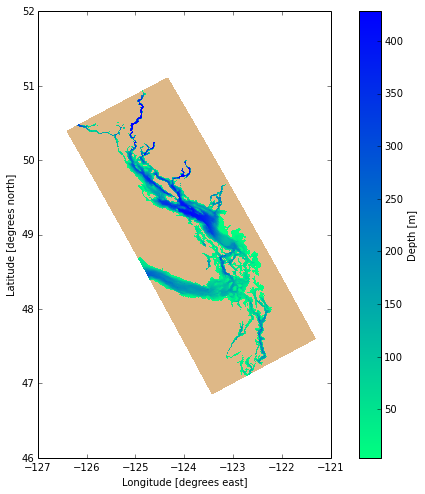This technique can be used to plot any NEMO results quantities on map coordinates because all of the NEMO netCDF output files contain nav_lat and nav_lon variables.

Not surprisingly, we can use plt.axis() to zoom in to a region of the domain in map coordinates too - just give the coordinates to zoom to in longitude and latitude instead of grid indices.

In :
fig, ax = plt.subplots(1, 1, figsize=(10, 8))
aspect = viz_tools.set_aspect(ax, coords='map', lats=lats)
cmap = plt.get_cmap('winter_r')
mesh = ax.pcolormesh(lons[:], lats[:], bathy[:], cmap=cmap)
cbar = fig.colorbar(mesh)
plt.axis((-123.5, -122.5, 48, 49))

ax.set_xlabel('{longitude.long_name} [{longitude.units}]'.format(longitude=lons))
ax.set_ylabel('{latitude.long_name} [{latitude.units}]'.format(latitude=lats))
cbar.set_label('{depth.long_name} [{depth.units}]'.format(depth=bathy))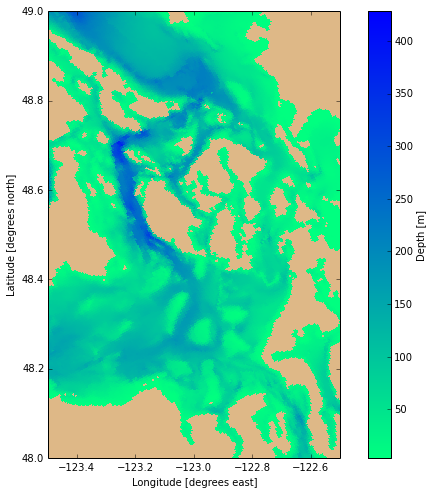## Saving Plots As Image Files and Displaying Image Files in Notebooks¶

Saving plots is as simple as calling the savefig() method of the fig object. The type of image file to create is determined from the extension of the file name you pass to savefig() or it can be specified explicitly via the format argument.

In :
fig.savefig('bathy.png')


PNG is a good format choice for graphs because it renders straight lines and text more clearly than JPG while still doing a good job on smooth transitions across colour changes.

For publications using LaTex chose EPS or PDF.

The following code produces a list of all of the image file formats that may be supported.

In :
import matplotlib.backend_bases
canvas = matplotlib.backend_bases.FigureCanvasBase(fig)
canvas.filetypes

Out:
{'eps': 'Encapsulated Postscript',
'jpeg': 'Joint Photographic Experts Group',
'jpg': 'Joint Photographic Experts Group',
'pdf': 'Portable Document Format',
'pgf': 'LaTeX PGF Figure',
'png': 'Portable Network Graphics',
'ps': 'Postscript',
'raw': 'Raw RGBA bitmap',
'rgba': 'Raw RGBA bitmap',
'svg': 'Scalable Vector Graphics',
'svgz': 'Scalable Vector Graphics',
'tif': 'Tagged Image File Format',
'tiff': 'Tagged Image File Format'}

Image files can also be displayed in notebooks with code like this:

In :
from IPython.display import Image
Image('bathy.png')

Out: